# VBA Range

## VBA Cells

The Cells keyword allows VBA to interact with cells in the worksheet.

    'Cells([row],[column])
Cells(1,1) = 10
Cells(2,1) = 20
Cells(3,1) = 30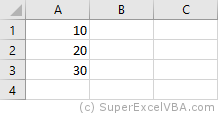As well as entering values, we can also read values:

    VarCell = Cells(1,1)
MsgBox VarCell


We can also perform operations, in the same way that can be done with variables:

    Cells(1,3) = Cells(1,1) + Cells(2,1)


Note that the final result of the above example will be available in cell "C1" of the active worksheet.

## VBA Range

Range is a command to represent intervals, containing one or more cells.

In order to establish a Range it is necessary to establish an initial cell and an end cell.

This can be done by using Cells:

    'Range([initial cell],[end cell])
Range(Cells(1,1),Cells(2,3)) = 7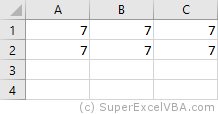You can also set a Range from a String notation.

    Range("A1:C2") = 10


In this notation, each cell is represented by a letter, referring to its column, and a number, referring to its row (E.g. "A1"). We establish with a colon : the identification of the initial cell and the end cell:

### VBA Range Object

We can perform other more complex actions with Range:

    Range("A1:B1").Merge            'Merge the "A1" and "B1" cells
Range("A1").Value ="Storage"    'Inserts a text in "A1"
Range("A2").Formula = "=10-5"   'Inserts a formula into "A2"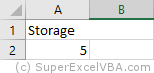Everything that comes after the dot (.) after the keyword Range is a Method or a Property.

• Method (like .Merge) is what performs an action.
• Property (like .Value) is what assigns (or has) a value or access other objects.

Throughout this tutorial we will describe the most useful Methods and Properties for the day-to-day business operations.

At the same time, everything that accepts a Method or a Property is an Object.

Therefore, we can access from the object Range the Property .Value, for example, to copy values from one range to another:

    Range("C1:D3").Value = Range("A1:B3").Value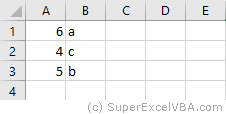Objects, Methods, and Properties are broad concepts present in other programming languages. The study on this site will focus on the practical use in VBA.

#### VBA Set

Objects in VBA are only associated with variables preceded by the Set statement.

    Set Rng = Range("B2:B7")
Rng.Value = 0 'this will set the value 0 from "B2" to "B7"


For objects there is the Object data type.

Advantages of associating a Range with a variable using Set:

• Memory loaded variables have faster processing
• Simplification of the code

### VBA Range Cells

It is possible to access cells within a Range using Cells.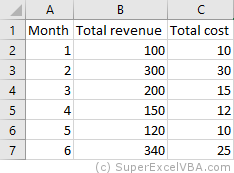MsgBox Range("B2:C7").Cells(3,2) 'This will display the value of cell "C4"


Cells is a property of Range.

The Cells reference will be based on the defined Range (in this case "B2" would be equivalent to Cells(1,1)).

### Worksheets Range

The Range keyword will always refer to a Worksheets. If there is no specification, it will refer to the active worksheet (ActiveSheet).

    Worksheets("Sheet1").Range("A1") 'Refers to the inactive worksheet "Sheet1"
Range("A1") 'Refers to the active worksheet "SuperExcelVBA"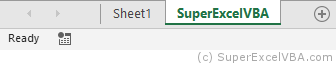Range in this example is a property of Worksheets.

We can specify the active worksheet with .Activate method.

    Worksheets("Sheet1").Activate 'Sheet1 will become the active worksheet


It is recommended to specify the worksheet whenever there is more than one in the same file.

You can explicitly reference the active worksheet by using the ActiveSheet command.

    Range("A1") = "Active Sheet"
ActiveSheet.Range("A1") = "Active Sheet" 'Same result as above
MsgBox ActiveSheet.Name 'Returns the name of the active sheet


You can also specify the worksheet according to its position in the file:

    Worksheets(1).Range("A1") 'Refers to the first worksheet in the order that appears in Excel ("Sheet1")
Worksheets(2).Range("A1") 'Refers to the second worksheet in the order that appears in Excel ("SuperExcelVBA")


Thus, we can refer to the same worksheet by both ways:

    Worksheets("Sheet1").Range("A1")
Worksheets(1).Range("A1")


Note that the first way will require changes in the code if there is a change in the name of the worksheet. In the second way, the change will be necessary if the worksheet changes position.

### WorkBook Range

In the same way that a Range always refers to a Worksheet, a Worksheet always refers to a Workbook (Excel file).

Although it is common to work with only one workbook opened, referencing the Workbook is a good practice if there is an interaction with another file.

    WorkBooks("Example.xlsm").Worksheets("Sheet1").Range("A1")
WorkBooks("Tutorial.xlsm").Worksheets("SuperExcelVBA").Range("A1")


The Worksheets in the example are a property of WorkBooks.

You need to specify both the file name and the file extension (E.g. .xlsm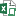) when you use WorkBooks.